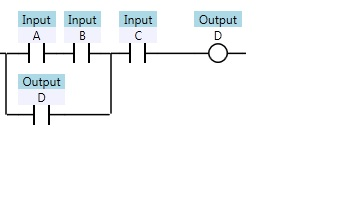Ladder diagrams are specialized schematics commonly used to document industrial control logic systems. They are called “ladder” diagrams because they resemble a ladder, with two vertical rails (supply power) and as many “rungs” (horizontal lines) as there are control circuits to represent.In this session we are going to discuss about basic ladder logic programs If we wanted to draw a simple ladder diagram showing a lamp that is controlled by a hand switch, it would look like this### Digital logic functions

We can construct simply logic functions for our hypothetical lamp circuit, using multiple contacts, and document these circuits quite easily and understandably with additional rungs to our original “ladder.” If we use standard binary notation for the status of the switches and lamp (0 for not actuated or de-energized; 1 for actuated or energized), a truth table can be made to show how the logic works:

#### OR GATENow, the lamp will come on if either contact A or contact B is actuated, because all it takes for the lamp to be energized is to have at least one path for current from wire L1 to wire 1. What we have is a simple OR logic function, implemented with nothing more than contacts and a lamp.

#### AND GATENow, the lamp energizes only if contact A and contact B are simultaneously actuated. A path exists for current from wire L1 to the lamp (wire 2) if and only if both switch contacts are closed.

#### NOT GATENow, the lamp energizes if the contact is not actuated, and de-energizes when the contact is actuated.

####If we take our OR function and invert each “input” through the use of normally-closed contacts, we will end up with a NAND function. In a special branch of mathematics known as Boolean algebra, this effect of gate function identity changing with the inversion of input signals is described by DeMorgan’s Theorem

The lamp will be energized if either contact is unactuated. It will go out only if both contacts are actuated simultaneously.

#### NOR GATELikewise, if we take our AND function and invert each “input” through the use of normally-closed contacts, we will end up with a NOR function:

If we wish to invert the output of any switch-generated logic function, we must use a relay with a normally-closed contact. For instance, if we want to energize a load based on the inverse, or NOT, of a normally-open contact, we could do this:

We will call the relay, “control relay 1,” or CR1. When the coil of CR1 (symbolized with the pair of parentheses on the first rung) is energized, the contact on the second rung opens, thus de-energizing the lamp. From switch A to the coil of CR1, the logic function is non inverted. The normally-closed contact actuated by relay coil CR1 provides a logical inverter function to drive the lamp opposite that of the switch’s actuation status.

Applying this inversion strategy to one of our inverted-input functions created earlier, such as the OR-to-NAND, we can invert the output with a relay to create a non inverted function:Scroll to Top